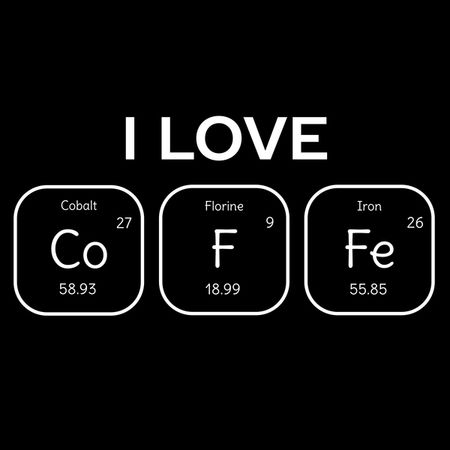Calculated Coffee Model
by: Sheila Stevenson (over 5 years ago)

Description

A polynomial function can be used to model a real-world situation.  The behavior of the graphs of polynomial functions of different degrees can suggest what type of polynomial function will best fit the data.  Students have studied linear, quadratic, and cubic polynomial functions and use graphing technology for a regression analysis.  In this project, iSense and Vernier Graphical Analysis is used to collect data for interpretation.

The question today is: Will your coffee stay warmer if you add milk?

(Optional: Have a class discussion with student's predicting the answer to the question of the day.)

Using the Vernier probe, three different cups of coffee temperatures were measured over a period of 180 seconds.  The data sets are: black (no soy milk), milk1 (1/4 cup soy milk), and milk2 (1/8 cup soy milk).  This data has been uploaded for students to analyze.

Questions for students:

1. Describe the rate of change in context for this data set.

2. What polynomial function will best fit this data set?

3. Using Analysis Tools, draw the line of best fit, selecting one data set at a time.  Write the function model for each cup of coffee and its corresponding R squared value.  Which cup of coffee cools at the slowest rate?

4. Using graphing technology, graph the three function models to verify your answer in Question 3. What is the meaning of the y-intercepts? What is the meaning of the point(s) of intersection?

5. Extra Credit: Read the article "The Physics of Coffee and Cream" using the resource link below.  What is the main reason for the results you obtained from the data set and the polynomial function stated in this article?Resource: The Physics of Coffee and Cream

http://modernistcuisine.com/2012/12/the-physics-of-coffee-cream/

Data source: Vernier Graphical Analysis completed on 11/16/17 and the data was uploaded into this iSense project.

Data SetsSheila Stevenson blackcoffeeSheila Stevenson milkcoffee2Sheila Stevenson milkcoffee1
Fields
Name Units Type
Data Set 1:Time(s)
None
Number
Data Set 1:Temperature(°C)
None
Number
Formula Fields
Name Units Type
Temperature
Celsius
Number
Time
seconds
Number
Contribute Data
Media

## Calculated Coffee Model

Project #3334 on iSENSEProject.org

Description

A polynomial function can be used to model a real-world situation.  The behavior of the graphs of polynomial functions of different degrees can suggest what type of polynomial function will best fit the data.  Students have studied linear, quadratic, and cubic polynomial functions and use graphing technology for a regression analysis.  In this project, iSense and Vernier Graphical Analysis is used to collect data for interpretation.

The question today is: Will your coffee stay warmer if you add milk?

(Optional: Have a class discussion with student's predicting the answer to the question of the day.)

Using the Vernier probe, three different cups of coffee temperatures were measured over a period of 180 seconds.  The data sets are: black (no soy milk), milk1 (1/4 cup soy milk), and milk2 (1/8 cup soy milk).  This data has been uploaded for students to analyze.

Questions for students:

1. Describe the rate of change in context for this data set.

2. What polynomial function will best fit this data set?

3. Using Analysis Tools, draw the line of best fit, selecting one data set at a time.  Write the function model for each cup of coffee and its corresponding R squared value.  Which cup of coffee cools at the slowest rate?

4. Using graphing technology, graph the three function models to verify your answer in Question 3. What is the meaning of the y-intercepts? What is the meaning of the point(s) of intersection?

5. Extra Credit: Read the article "The Physics of Coffee and Cream" using the resource link below.  What is the main reason for the results you obtained from the data set and the polynomial function stated in this article?Resource: The Physics of Coffee and Cream

http://modernistcuisine.com/2012/12/the-physics-of-coffee-cream/

Data source: Vernier Graphical Analysis completed on 11/16/17 and the data was uploaded into this iSense project.

Fields
Name Units Type of Data
Data Set 1:Time(s)
None
Number
Data Set 1:Temperature(°C)
None
Number
Formula Fields
Name Units Type of Data
Temperature
Celsius
Number
Time
seconds
Number

Our Data
Name(s): ______________________________________
Date: _________________________________________

 Data Set 1:Time(s) Data Set 1:Temperature(°C)